# NCERT solutions for Class 10 Maths: Chapter 13 Surface Area and Volume Exercise 13.2

In this page we have NCERT solutions for Class 10 Maths: Chapter 13 Surface Area and Volume for Exercise 13.3 on pages 251 and 252. Hope you like them and do not forget to like , social_share and comment at the end of the page.

This exercise is based on the volume of an object formed by combining any two of the basic solids, namely, cuboid, cone, cylinder, sphere and hemisphere
Formula to used
The total Volume of the any new solid is the sum of the Volume of each of the individual parts.
Volume of new solid = Volume of One part + Volume of Second part + Volume of Third part
Volume of Common Shapes
Volume of cone
$=\frac {1}{3}\pi r^2h$
Volume of Sphere
$=\frac {4}{3} \pi r^3$
Volume of Cylinder
$=\pi r^2 h$
Unless stated otherwise, take $\pi = \frac {22}{7}$.

## Surface Area and Volume Exercise 13.3

Question 1
A metallic sphere of radius 4.2 cm is melted and recast into the shape of a cylinder of radius 6 cm. Find the height of the cylinder.
Solution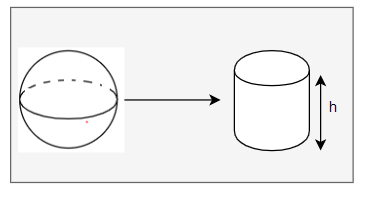Let h be the height then
Then , volume of cylinder =Volumne of Sphere
$\pi (6)^2 h =\frac {4}{3} \pi (4.2)^3$
then h =2.74 cm

Question 2
Metallic spheres of radii 6 cm, 8 cm and 10 cm, respectively, are melted to form a single solid sphere. Find the radius of the resulting sphere.
Solution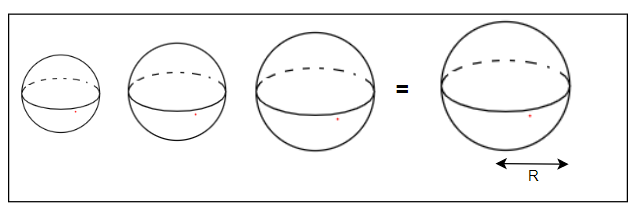Now
Volume of resulting sphere = Volume of 6 cm sphere + Volume of 8 cm sphere + Volume of 10 cm sphere
$\frac {4}{3} \pi R^3 = \frac {4}{3}\pi 6^3 + \frac {4}{3}\pi 8^3 + \frac {4}{3}\pi 10^3$
or
$R^3= 6^3 + 8^3 + 10^3$
$R^3=1728$
R=12 cm

Question 3
A 20 m deep well with diameter 7 m is dug and the earth from digging is evenly spread out to form a platform 22 m by 14 m. Find the height of the platform.
Solution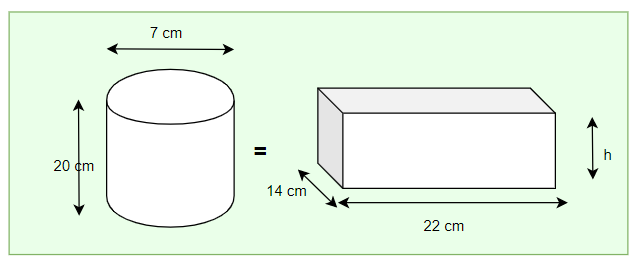Let h be the height of platform
Now
Volume Of well = Volume of the platform formed
$\pi (3.5)^2 20 = 22 \times 14 \times h$
h=2.5 m

Question 4
A well of diameter 3 m is dug 14 m deep. The earth taken out of it has been spread evenly all around it in the shape of a circular ring of width 4 m to form an embankment. Find the height of the embankment.
Solution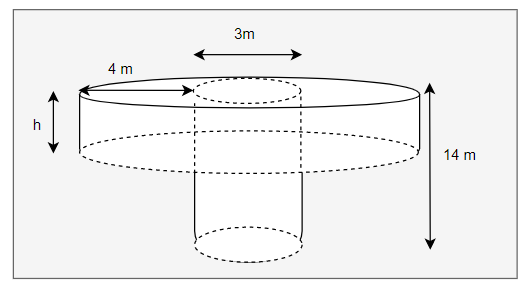Let h be the height of the embankment.
It is aparant from figue the embankment is a cylinderical right with inner radii= 1.5 m and outer radii = 5.5 m
Now
Volume Of well = Volume of the embankment formed
$\pi \times (1.5)^2 \times 14 = \pi \times h \times (5.5^2 - 1.5^2)$
h=1.125 m

Question 5
A container shaped like a right circular cylinder having diameter 12 cm and height 15 cm is full of ice cream. The ice cream is to be filled into cones of height 12 cm and diameter 6 cm, having a hemispherical shape on the top. Find the number of such cones which can be filled with ice cream.
Solution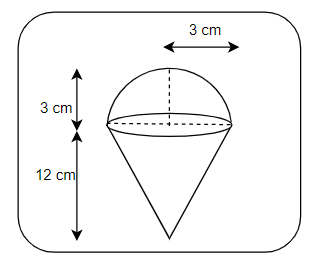Radius of Cone(r) = 6/2 = 3 cm
Height of cone(h)= 12 cm
Radius of hemispherical shape on the top(r) = 3cm
Volume of One cone icecream = $\frac {1}{3} \pi r^2 h + \frac {2}{3} \pi r^3 = \frac {1}{3} \pi ( 108 + 54)$
Let n be the number of icecream cones filled
Then
Volume of Cylinder = n × Volume of One cone icecream
$\pi (6)^2 15 = \frac {n}{3} \pi ( 108 + 54)$
or n=10

Question 6
How many silver coins, 1.75 cm in diameter and of thickness 2 mm, must be melted to form a cuboid of dimensions 5.5 cm x 10 cm x 3.5 cm?
Solution
Let n be the silver coins needed
n × Volume of one silver coin= volume of cuboid
$n \times \pi \times (.875)^2 \times .2 = 5.5 \times 10 \times 3.5$
n=400

Question 7
A cylindrical bucket, 32 cm high and with radius of base 18 cm, is filled with sand. This bucket is emptied on the ground and a conical heap of sand is formed. If the height of the conical heap is 24 cm, find the radius and slant height of the heap.
Solution
Let r be the radius of the conical heap
Now Volume of cylinderical bucket = Volume of conical heap
$\pi \times (18)^2 \times 32 = \frac {1}{3} r^2 \times 24$
r=36 cm
Now slant height = $\sqrt {r^2 + h^2} = \sqrt {36^2 + 24^2} = 12 \sqrt {13}$ cm

Question 8
Water in a canal, 6 m wide and 1.5 m deep, is flowing with a speed of 10 km/h. How much area will it irrigate in 30 minutes, if 8 cm of standing water is needed?
Solution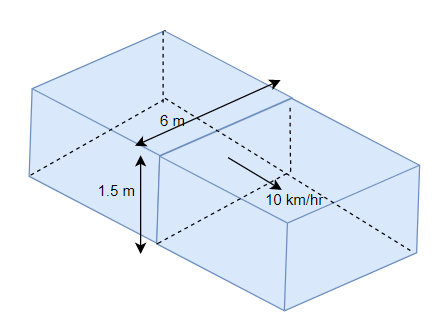Cross-section of the Canal = $6 \times 1.5 = 9 m^2$
Now Water speed is 10 km/hr = 1000/6 m/min
So volume of Water flowed in 1 min = $9 \times \frac {1000}{6}$
Therefore Volume of water in 30 min = $9 \times \frac {1000}{6} \times 30=45000 m^3$

Let A be the Area irrigated then
Then
$A \times .08 = 45000$
A=562500 m2

Question 9
A farmer connects a pipe of internal diameter 20 cm from a canal into a cylindrical tank in her field, which is 10 m in diameter and 2 m deep. If water flows through the pipe at the rate of 3 km/h, in how much time will the tank be filled?
Solution
Cross-section of the pipe = $\pi \times (.1)^2 = .01 \pi m^2$
Speed of water = 3km/h = 3000/60 m/min = 50 m/min
Let t be the time time taken in min, then Volume of water out of pipe
$= .01 \pi \times 50 \times t = .5 t \pi m^2$

Now Volume of cylindrical tank= $\pi (5)^2 2= 50 \pi m^2$
Now Volume of water out of pipe=Volume of cylindrical tank
$.5 t \pi=50 \pi$
t= 100 min

## Summary

1. Class 10 Maths NCERT solutions for Surface Area and Volume Exercise 13.3 has been prepared by Expert with utmost care. If you find any mistake.Please do provide feedback on mail.You can download this as pdf
2. This chapter 13 has total 5 Exercise 13.1 ,13.2,13.3 ,13.4 and 13.5. This is the Third exercise in the chapter.You can explore previous exercise of this chapter by clicking the link below

• Notes
• Assignments
• NCERT Solutions
• NCERT Solution Surface Area and Volume Class10 Exercise 13.1
• NCERT Solution Surface Area and Volume Class10 Exercise 13.2
• NCERT Solution Surface Area and Volume Class10 Exercise 13.3Go back to Class 10 Main Page using below links

### Practice Question

Question 1 What is $1 - \sqrt {3}$ ?
A) Non terminating repeating
B) Non terminating non repeating
C) Terminating
D) None of the above
Question 2 The volume of the largest right circular cone that can be cut out from a cube of edge 4.2 cm is?
A) 19.4 cm3
B) 12 cm3
C) 78.6 cm3
D) 58.2 cm3
Question 3 The sum of the first three terms of an AP is 33. If the product of the first and the third term exceeds the second term by 29, the AP is ?
A) 2 ,21,11
B) 1,10,19
C) -1 ,8,17
D) 2 ,11,20